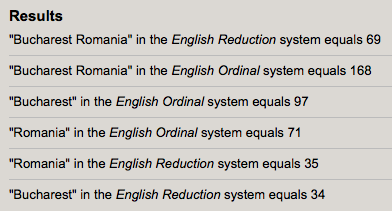## Friday, October 30, 2015

### 26 42 44 96 | Romanian Nightclub Explodes, 26 Killed, October 30/31, 2015

First, notice the coordinates of Bucharest, Romania, where 26 people were reportedly killed.

Kill = 2+9+3+3 = 17
Kill = 11+9+3+3 = 26
Kill = 11+9+12+12 = 44

Also notice the 112 in the background.  Remember, 112 is the alternate for 911.

This bombing/explosive happened in a Romanian Nightclub.

Romanian = 9+6+4+1+5+9+1+5 = 40
Nightclub = 5+9+7+8+2+3+3+3+2 = 42
Romanian Nightclub = 82

Romanian = 18+15+13+1+14+9+1+14 = 85
Nightclub = 14+9+7+8+20+3+12+21+2 = 96
Romanian Nightclub = 1811.1.